# Problem 2If change the beam to the one shown below, will all your calculation in Problem 1 change? Please plot V and Mdiagram and use at least one sentence to explain based on your plot. Note: No need to calculate the stresses inProblem 1.2500 lb/ftB6000 lb6 ft

Question
33 views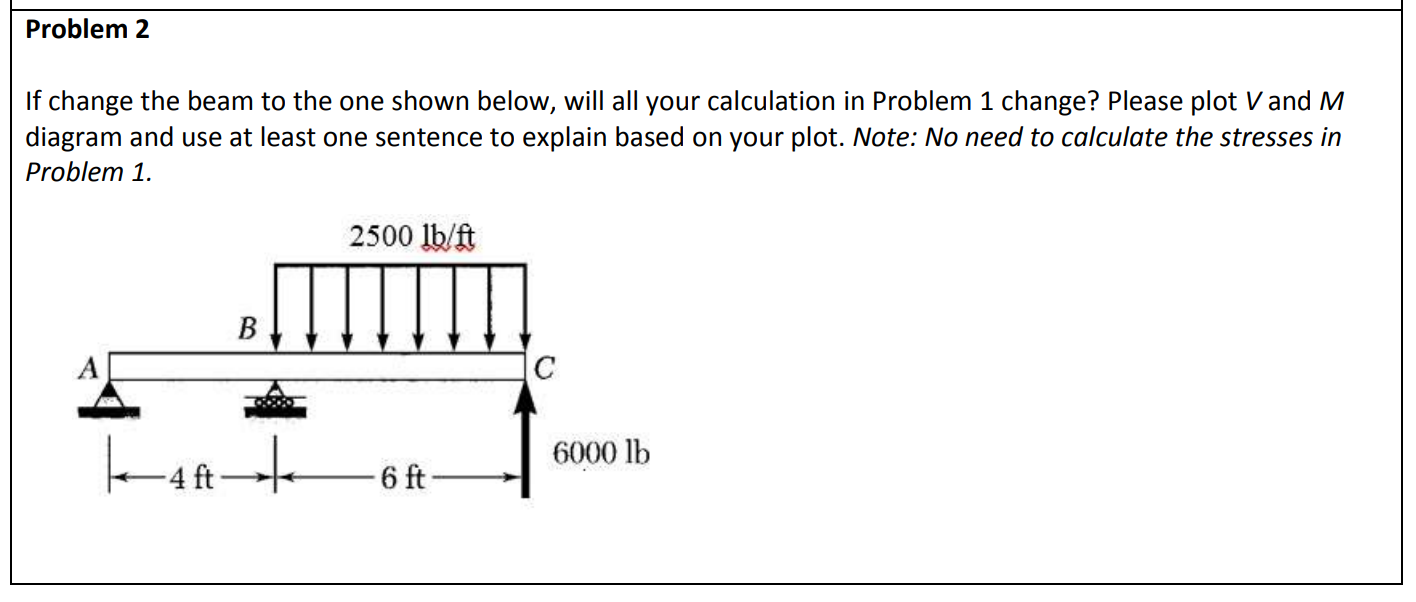help_outlineImage TranscriptioncloseProblem 2 If change the beam to the one shown below, will all your calculation in Problem 1 change? Please plot V and M diagram and use at least one sentence to explain based on your plot. Note: No need to calculate the stresses in Problem 1. 2500 lb/ft B 6000 lb 6 ft fullscreen
check_circle

Step 1

Given:

The beam is shown below,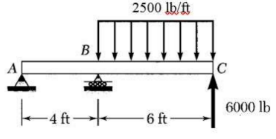Step 2

Calculate the reaction on the beam.

Take summation of moment at A is 0.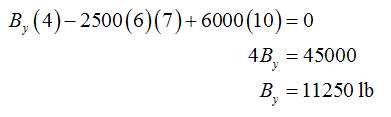Take summation of vertical forces is 0.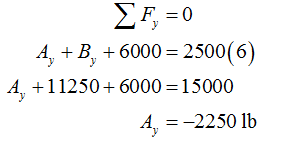Step 3

The V&M diagram is shown below,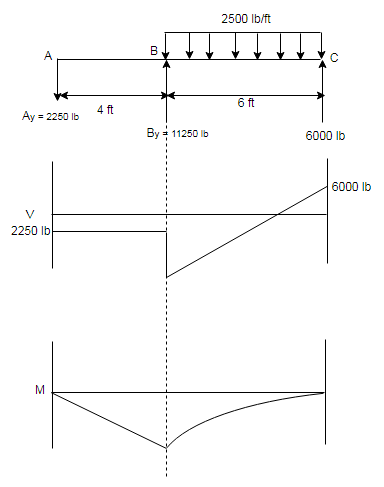Figure (1)

...

### Want to see the full answer?

See Solution

#### Want to see this answer and more?

Solutions are written by subject experts who are available 24/7. Questions are typically answered within 1 hour.*

See Solution
*Response times may vary by subject and question.
Tagged in